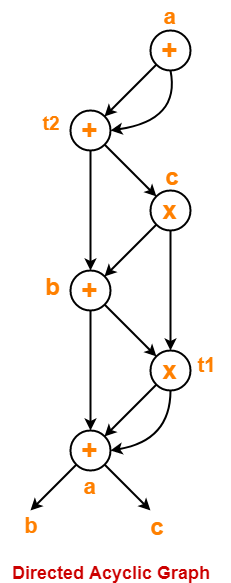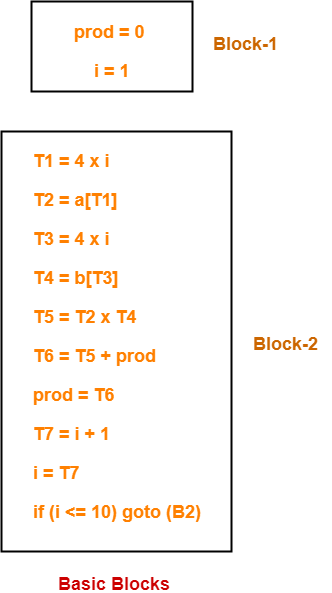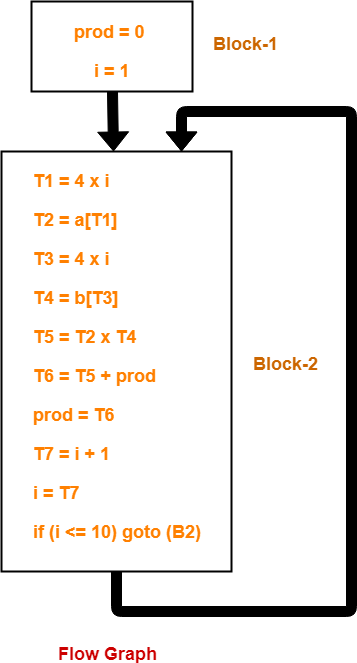# Miscellaneous Problems in Compiler Design

## Three Address Code | DAGs | Basic Blocks & Flow Graphs-

Before you go through this article, make sure that you have gone through the previous articles on-

In this article, we will solve Miscellaneous Problems based on these Concepts.

## Problem-01:

Generate three address code for the following code-

c = 0

do

{

if (a < b) then

x++;

else

x–;

c++;

} while (c < 5)

## Solution-

Three address code for the given code is-

1. c = 0
2. if (a < b) goto (4)
3. goto (7)
4. T1 = x + 1
5. x = T1
6. goto (9)
7. T2 = x – 1
8. x = T2
9. T3 = c + 1
10. c = T3
11. if (c < 5) goto (2)

## Problem-02:

Generate three address code for the following code-

while (A < C and B > D) do

if A = 1 then C = C + 1

else

while A <= D

do A = A + B

## Solution-

Three address code for the given code is-

1. if (A < C) goto (3)
2. goto (15)
3. if (B > D) goto (5)
4. goto (15)
5. if (A = 1) goto (7)
6. goto (10)
7. T1 = c + 1
8. c = T1
9. goto (1)
10. if (A <= D) goto (12)
11. goto (1)
12. T2 = A + B
13. A = T2
14. goto (10)

## Problem-03:

Generate three address code for the following code-

switch (ch)

{

case 1 : c = a + b;

break;

case 2 : c = a – b;

break;

}

## Solution-

Three address code for the given code is-

if ch = 1 goto L1

if ch = 2 goto L2

L1:

T1 = a + b

c = T1

goto Last

L2:

T1 = a – b

c = T2

goto Last

Last:

## Problem-04:

Construct a DAG for the following three address code-

1. a = b + c
2. t1 = a x a
3. b = t1 + a
4. c = t1 x b
5. t2 = c + b
6. a = t2 + t2

## Solution-

Directed acyclic graph for the given three address code is-## Problem-05:

Consider the following code-

prod = 0 ;

i = 1 ;

do

{

prod = prod + a[ i ] x b[ i ] ;

i = i + 1 ;

} while (i <= 10) ;

1. Compute the three address code.
2. Compute the basic blocks and draw the flow graph.

## Solution-

### Part-01:

Three address code for the given code is-

prod = 0

i = 1

T1 = 4 x i

T2 = a[T1]

T3 = 4 x i

T4 = b[T3]

T5 = T2 x T4

T6 = T5 + prod

prod = T6

T7 = i + 1

i = T7

if (i <= 10) goto (3)

### Step-01:

We identify the leader statements as-

• prod = 0 is a leader because first statement is a leader.
• T1 = 4 x i is a leader because target of conditional or unconditional goto is a leader.

### Step-02:

The above generated three address code can be partitioned into 2 basic blocks as-### Step-03:

The flow graph is-To gain better understanding about these Miscellaneous Problems,

Watch this Video LectureNext Article- Code Optimization

Get more notes and other study material of Compiler Design.

Watch video lectures by visiting our YouTube channel LearnVidFun.

SummaryArticle Name
Miscellaneous Problems in Compiler Design
Description
Practice Problems on Three Address Code, Directed Acyclic Graphs and Basic Blocks & Flow Graphs in Compiler Design. Questions from Three Address Code, Directed Acyclic Graphs and Basic Blocks & Flow Graphs are frequently asked in compiler design.
Author
Publisher Name
Gate Vidyalay
Publisher Logo